Question

# What would the result be if you ran the following code: function makeAdder(x) { return function(y)...

What would the result be if you ran the following code:

return function(y) {
return x + y;
};
}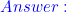```12
17```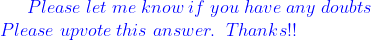#### Earn Coins

Coins can be redeemed for fabulous gifts.

Similar Homework Help Questions
• ### Which of the following replacement code fragments will fix the code below? console.log(square); console.log(square(5)); var square...

Which of the following replacement code fragments will fix the code below? console.log(square); console.log(square(5)); var square = function(n) {   return n * n; } A.) alert(square); alert(square(5)); var square = function(n) {   return n * n;  } B.) console.log(square); console.log(square(5)); var function square = function(n) {   return n * n;  } C.) console.log(square); console.log(square(5)); function square = function(n) {   return n * n;  } D.) console.log(square); console.log(square(5)); function square(n) {   return n * n;  }

• ### QUESTION 1 What will be displayed as a result of executing the following code? int   x...

QUESTION 1 What will be displayed as a result of executing the following code? int   x = 5, y = 20; x += 32; y /= 4; cout <<"x = " << x <<"y = " << y; A. x = 32, y = 4 B. x = 9, y = 52 C. x = 37, y = 5 D. x = 160, y = 80 8 points    QUESTION 2 What will be the displayed when the following code...

• ### A C function fun has the following code body: *p -d; return x-c; The IA32 code implementing this ...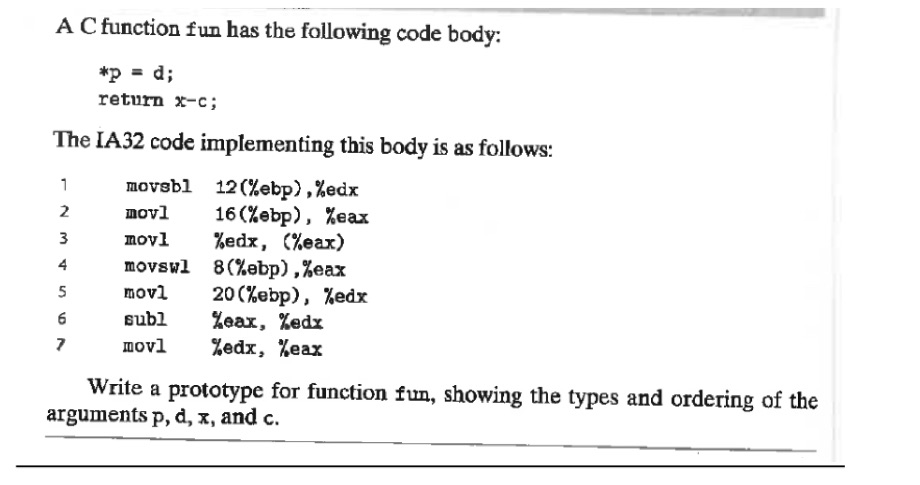A C function fun has the following code body: *p -d; return x-c; The IA32 code implementing this body is as follows 12 (%ebp) ,%eax 16(%ebp) , movabl nov1 nov1 %edx ,(%eax) movsw1 8(%ebp),%eax movl 20 (ebp), %edx subi %eax,%edx movi %edx, %eax 2 %eax 4 7 Write a prototype for function fun, showing the types and ordering of the arguments p, d, x, and c. A C function fun has the following code body: *p -d; return x-c; The...

• ### 2. What is the value of x alter the following code is executed # include<iostream> using...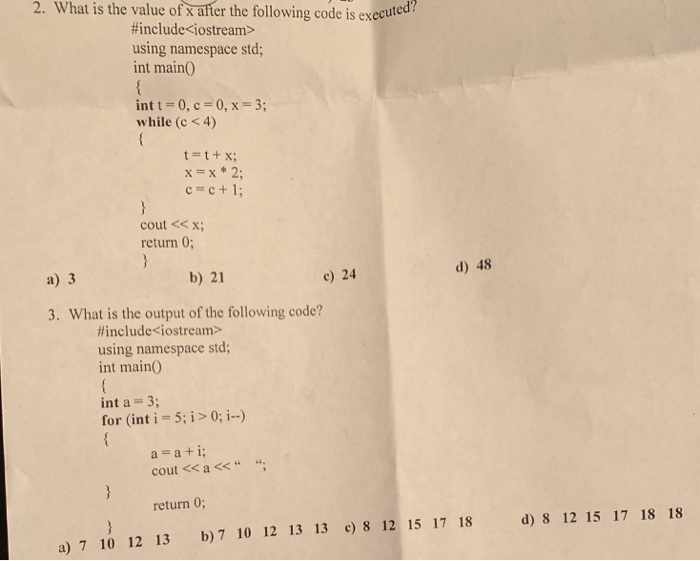2. What is the value of x alter the following code is executed # include<iostream> using namespace std; int main int t-0, c- 0,x-3; while (c < 4) t=t+x; cout << x return 0; a) 3 b) 21 c) 24 d) 48 3. What is the output of the following code? # include<iostream> using namespace std; int main0 int a- 3; for (int i 5; i >0; i) a a+i cout << a << “ “ return 0; d) 8...

• ### 8. (10%) Consider the following JavaScript program: // The main program var x,y; function f10{ var...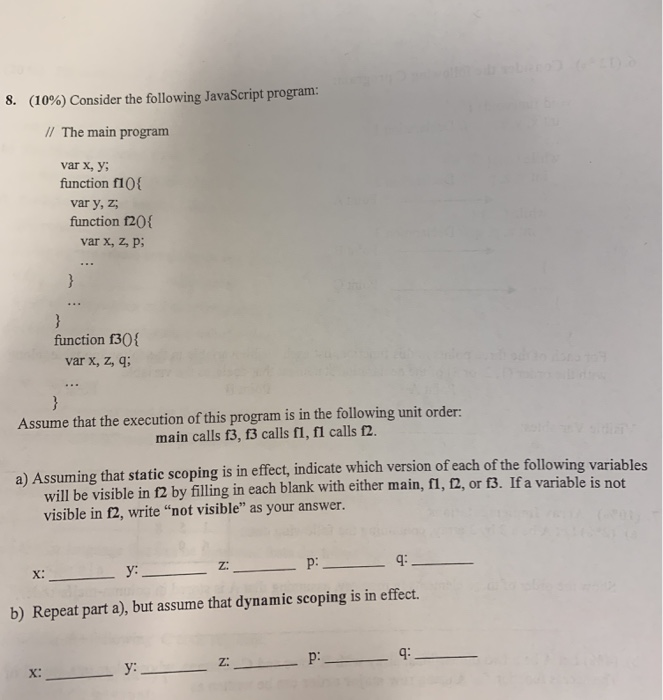8. (10%) Consider the following JavaScript program: // The main program var x,y; function f10{ var y, z; function f20{ var x, Z, P; function f30{ var x, z, ; Assume that the execution of this program is in the following unit order: main calls f3, f3 calls f1, f1 calls 12. a) Assuming that static scoping is in effect, indicate which version of each of the following variables will be visible in f2 by filling in each blank with...

• ### def max_of_two( x, y): ifx>y: return x returny a. Write a Python function called max_of_three( x,...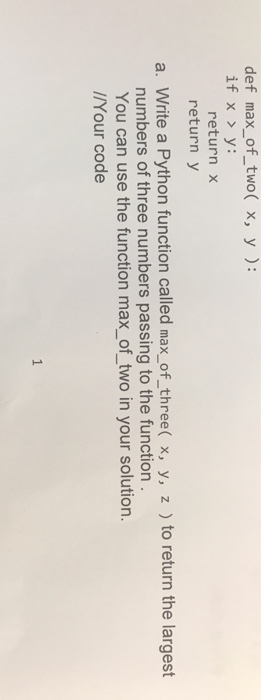def max_of_two( x, y): ifx>y: return x returny a. Write a Python function called max_of_three( x, y, z ) to return the largest numbers of three numbers passing to the function You can use the function max_of_two in your solution. /Your code

• ### 1. var s = "A red boat"; var a = s.split(" "); what is the value...

1. var s = "A red boat"; var a = s.split(" "); what is the value of a? var b = [9, 3, 2, 1, 3, 7]; var c = b.slice(2, 5); What is the value of c? var d = c.concat(a); alert(d.join("**")); [ 'A', 'red' , 'boat'] [ 2 , 1, 3] 2**1**3**A**red**boat [ 'A', 'red' , 'boat'] [ 1 , 3, 7] 8**A**red**boat [ 'A', 'red' , 'boat'] [ 3 , 2, 1] A**red**boat [ 'A', 'red' ,...

• ### 8. Given the following code fragment and function definition, what is(are) the output(s)? int funct (int...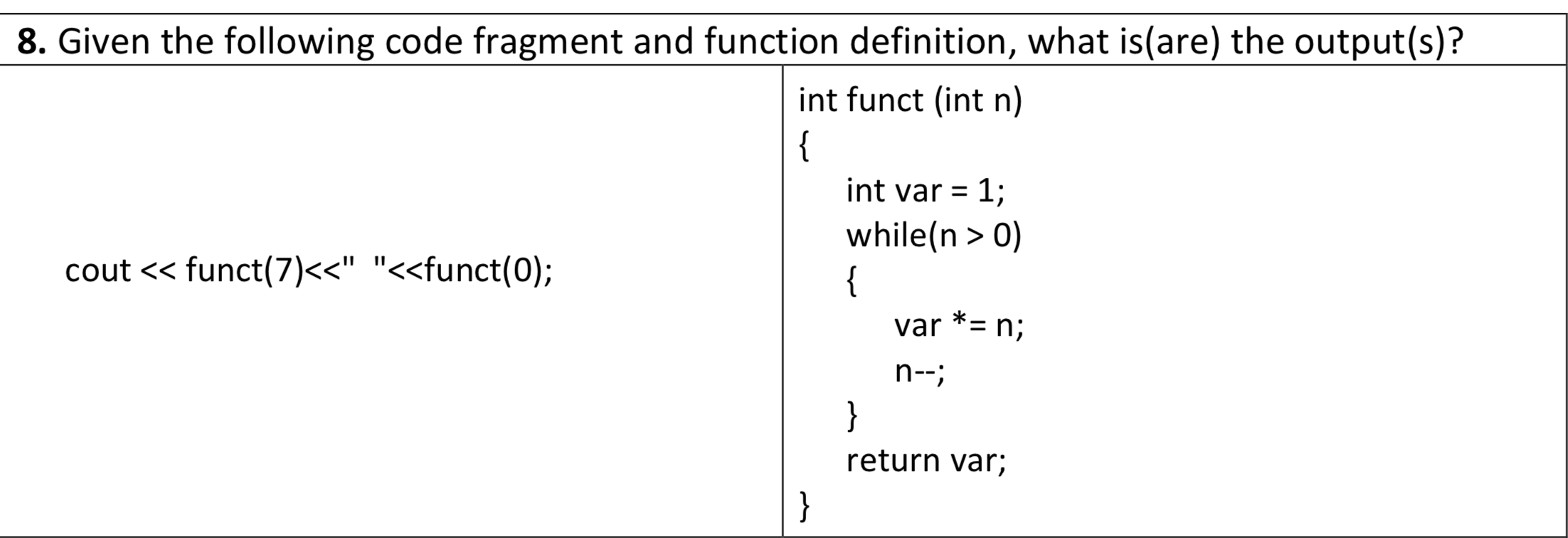8. Given the following code fragment and function definition, what is(are) the output(s)? int funct (int n) int var = 1; while(n > 0) cout << funct(7)<<" "<<funct(0); var *= n; n--; return var;

• ### The following JavaScript code will place the result of a multiplication in the result text box....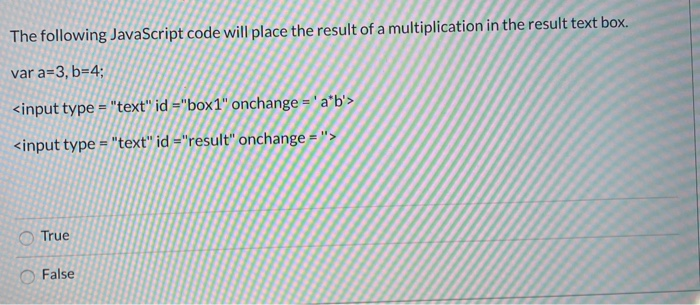The following JavaScript code will place the result of a multiplication in the result text box. var a=3, b=4; <input type = "text" id ="box1" onchange = 'a'b'> <input type="text" id ="result" onchange = True False

• ### Consider the following function: def w(x, y) : z = x + y return z What...

Consider the following function: def w(x, y) : z = x + y return z What is the function's name? Group of answer choices w x y z